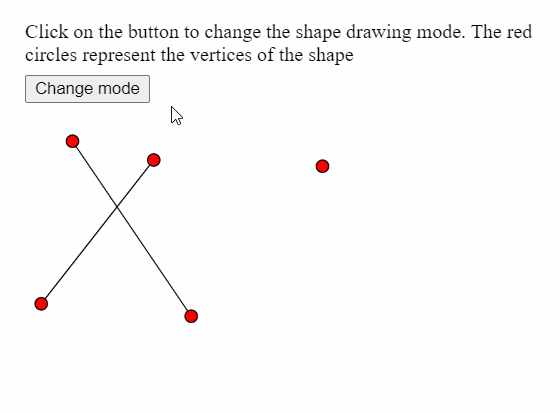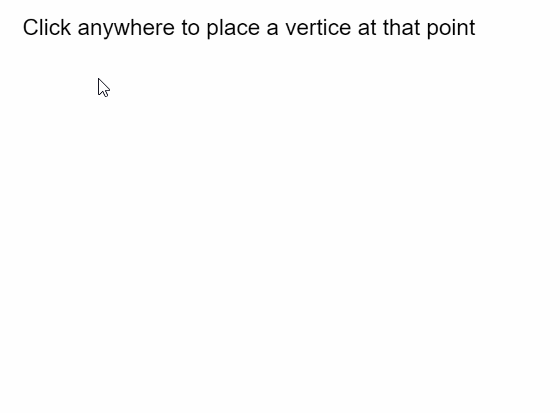# p5.js | vertex() Function

The vertex() function in p5.js is used to specify the coordinates of the vertices used to draw a shape. It can only be used with the beginShape() and endShape() functions to make various shapes and curves like points, lines, triangles, quads and polygons.

Syntax:

`vertex( x, y )`

OR

`vertex( x, y, z, [u], [v] )`

Parameters: This function accepts five parameters as mentioned above and described below:

• x: It is a number that specifies the x-coordinate of the vertex.
• y: It is a number that specifies the y-coordinate of the vertex.
• z: It is a number that specifies the z-coordinate of the vertex.
• u: It is a number that specifies the u-coordinate of the texture of the vertex. It is an optional parameter.
• v: It is a number that specifies the v-coordinate of the texture of the vertex. It is an optional parameter.

The examples below illustrates the vertex() function in p5.js:

Example 1:

 `let currMode; ` ` `  `function` `setup() { ` `  ``createCanvas(400, 300); ` `  ``textSize(18); ` ` `  `  ``let shapeModes = [LINES, TRIANGLES, TRIANGLE_FAN,  ` `                    ``TRIANGLE_STRIP, QUADS]; ` `  ``let index = 0; ` `  ``currMode = shapeModes[index]; ` ` `  `  ``let helpText = createP( ` `    ```Click on the button to change the shape drawing mode. ` `    ``The red circles represent the vertices of the shape` ` `  ``); ` `  ``helpText.position(20, 0); ` ` `  `  ``let closeBtn = createButton(``"Change mode"``); ` `  ``closeBtn.position(20, 60); ` `  ``closeBtn.mouseClicked(() => { ` `    ``if` `(index < shapeModes.length) index++; ` `    ``else` `index = 0; ` `    ``currMode = shapeModes[index]; ` `  ``}); ` `} ` ` `  `function` `draw() { ` `  ``clear(); ` ` `  `  ``// Starting the shape using beginShape() ` `  ``beginShape(currMode); ` ` `  `  ``// Specifying all the vertices ` `  ``vertex(145, 245); ` `  ``vertex(50, 105); ` `  ``vertex(25, 235); ` `  ``vertex(115, 120); ` `  ``vertex(250, 125); ` ` `  `  ``// Ending the shape using endShape() ` `  ``endShape(); ` ` `  `  ``// Points for demonstration ` `  ``fill(``"red"``); ` `  ``circle(145, 245, 10); ` `  ``circle(50, 105, 10); ` `  ``circle(25, 235, 10); ` `  ``circle(115, 120, 10); ` `  ``circle(250, 125, 10); ` `  ``noFill(); ` `} `

Output:Example 2:

 `let vertices = []; ` ` `  `function` `setup() { ` `  ``createCanvas(400, 300); ` `  ``textSize(18); ` `  ``text(``"Click anywhere to place a vertice "``+ ` `       ``"at that point"``, 10, 20); ` `} ` ` `  `function` `mouseClicked() { ` `  ``// Update the vertices array with ` `  ``// current mouse position ` `  ``vertices.push({ x: mouseX, y: mouseY }); ` ` `  `  ``clear(); ` `  ``fill(``"black"``); ` `  ``text(``"Click anywhere to place a vertice "``+ ` `       ``"at that point"``, 10, 20); ` ` `  `  ``noFill(); ` ` `  `  ``// Draw shape using the current vertices array ` `  ``beginShape(); ` `  ``for` `(let i = 0; i < vertices.length; i++) ` `    ``vertex(vertices[i].x, vertices[i].y); ` `  ``endShape(CLOSE); ` ` `  `  ``fill(``"red"``); ` `  ``// Draw a circle at all the vertices ` `  ``for` `(let i = 0; i < vertices.length; i++) ` `    ``circle(vertices[i].x, vertices[i].y, 15); ` `} `

Output:Online editor: https://editor.p5js.org/

Reference: https://p5js.org/reference/#/p5/vertexMy Personal Notes arrow_drop_upIm listening

If you like GeeksforGeeks and would like to contribute, you can also write an article using contribute.geeksforgeeks.org or mail your article to contribute@geeksforgeeks.org. See your article appearing on the GeeksforGeeks main page and help other Geeks.

Please Improve this article if you find anything incorrect by clicking on the "Improve Article" button below.

Article Tags :

Be the First to upvote.

Please write to us at contribute@geeksforgeeks.org to report any issue with the above content.